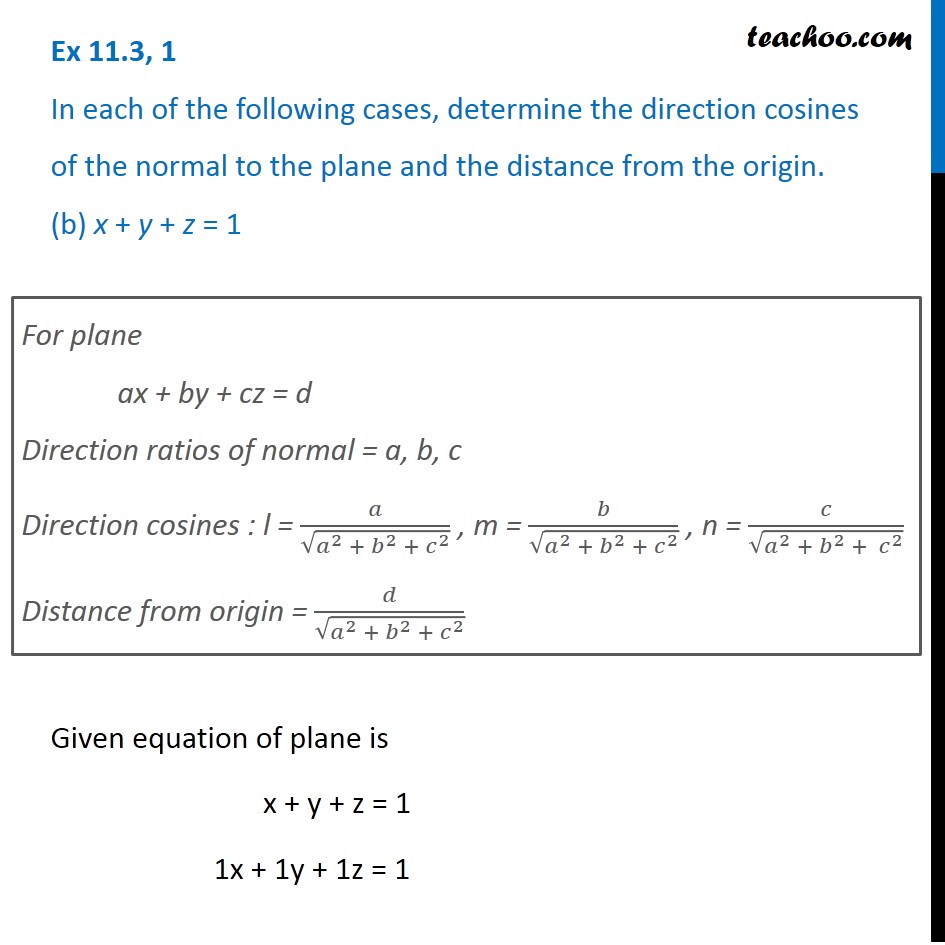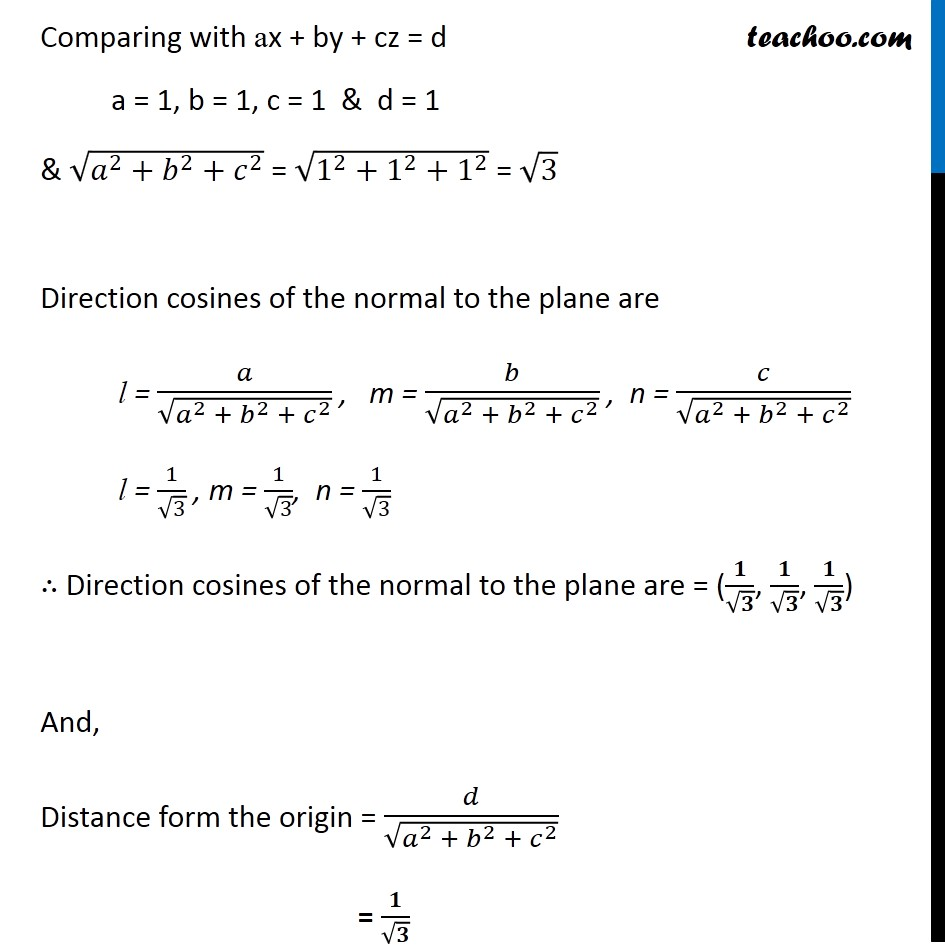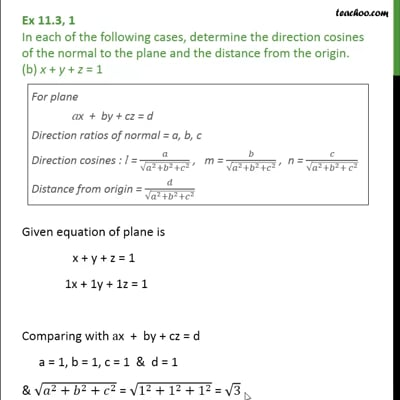Plane

Chapter 11 Class 12 Three Dimensional Geometry
Serial order wiseThis video is only available for Teachoo black users

Learn in your speed, with individual attention - Teachoo Maths 1-on-1 Class

### Transcript

Question 1 In each of the following cases, determine the direction cosines of the normal to the plane and the distance from the origin. (b) x + y + z = 1 For plane ax + by + cz = d Direction ratios of normal = a, b, c Direction cosines : l = 𝑎/√(𝑎^2 + 𝑏^2 +〖 𝑐〗^2 ) , m = 𝑏/√(𝑎^2 + 𝑏^2 + 𝑐^2 ) , n = 𝑐/√(𝑎^2 + 𝑏^2 + 𝑐^2 ) Distance from origin = 𝑑/√(𝑎^2 + 𝑏^2 + 𝑐^2 ) Given equation of plane is x + y + z = 1 1x + 1y + 1z = 1 Comparing with ax + by + cz = d a = 1, b = 1, c = 1 & d = 1 & √(𝑎^2+𝑏^2+𝑐^2 ) = √(1^2+1^2+1^2 ) = √3 Direction cosines of the normal to the plane are l = 𝑎/√(𝑎^2 + 𝑏^2 + 𝑐^2 ) , m = 𝑏/√(𝑎^2 + 𝑏^2 + 𝑐^2 ) , n = 𝑐/√(𝑎^2 + 𝑏^2 + 𝑐^2 ) l = 1/√3 , m = 1/√3, n = 1/√3 ∴ Direction cosines of the normal to the plane are = (𝟏/√𝟑, 𝟏/√𝟑, 𝟏/√𝟑) And, Distance form the origin = 𝑑/√(𝑎^2 + 𝑏^2 + 𝑐^2 ) = 𝟏/√𝟑